Normal view MARC view ISBD view

# Gravity : Newtonian, post-Newtonian, relativistic / Eric Poisson, University of Guelph, Clifford M. Will, University of Florida.

Material type:TextPublisher: c2014Description: xiv, 780 p., UKP 50.00 ill.; 25 cm.ISBN: 9781107032866 (hardback).DDC classification: 531/.1401 Other classification: SCI015000 Online resources: Cover image
Contents:
Machine generated contents note: Preface; 1. Foundations of Newtonian gravity; 2. Structure of self-gravitating bodies; 3. Newtonian orbital dynamics; 4. Minkowski spacetime; 5. Curved spacetime; 6. Post-Minkowskian theory: formulation; 7. Post-Minkowskian theory: implementation; 8. Post-Newtonian theory: fundamentals; 9. Post-Newtonian theory: system of isolated bodies; 10. Post-Newtonian celestial mechanics, astrometry and navigation; 11. Gravitational waves; 12. Radiative losses and radiation reaction; 13. Alternative theories of gravity; References; Index.
Summary: "This textbook explores approximate solutions to general relativity and their consequences. It offers a unique presentation of Einstein's theory by developing powerful methods that can be applied to astrophysical systems. Beginning with a uniquely thorough treatment of Newtonian gravity, the book develops post-Newtonian and post-Minkowskian approximation methods to obtain weak-field solutions to the Einstein field equations. The book explores the motion of self-gravitating bodies, the physics of gravitational waves, and the impact of radiative losses on gravitating systems. It concludes with a brief overview of alternative theories of gravity. Ideal for graduate courses on general relativity and relativistic astrophysics, the book examines real-life applications, such as planetary motion around the Sun, the timing of binary pulsars, and gravitational waves emitted by binary black holes. Text boxes explore related topics and provide historical context, and over 100 exercises present challenging tests of the material covered in the main text"--
Item type Current location Call number Status Date due Barcode Item holds
Book Chennai Mathematical Institute
General Stacks
531.140 POI (Browse shelf) Available 9186
Total holds: 0
##### Browsing Chennai Mathematical Institute Shelves , Shelving location: General Stacks Close shelf browser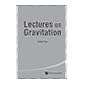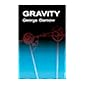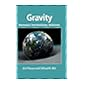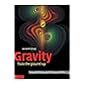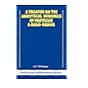531.113 LEI Nonlinear waves / 531.14 DAS Lectures on gravitation / 531.14 GAM Gravity / 531.140 POI Gravity : Newtonian, post-Newtonian, relativistic / 531.14 SCH Gravity from the ground up / 531.14 WHE A journey into gravity and spacetime / 531.163 WHI A treatise on the analytical dynamics of particles and rigid bodies : with an introduction to the problem of three bodies /

Includes bibliographical references and index.

Machine generated contents note: Preface; 1. Foundations of Newtonian gravity; 2. Structure of self-gravitating bodies; 3. Newtonian orbital dynamics; 4. Minkowski spacetime; 5. Curved spacetime; 6. Post-Minkowskian theory: formulation; 7. Post-Minkowskian theory: implementation; 8. Post-Newtonian theory: fundamentals; 9. Post-Newtonian theory: system of isolated bodies; 10. Post-Newtonian celestial mechanics, astrometry and navigation; 11. Gravitational waves; 12. Radiative losses and radiation reaction; 13. Alternative theories of gravity; References; Index.

"This textbook explores approximate solutions to general relativity and their consequences. It offers a unique presentation of Einstein's theory by developing powerful methods that can be applied to astrophysical systems. Beginning with a uniquely thorough treatment of Newtonian gravity, the book develops post-Newtonian and post-Minkowskian approximation methods to obtain weak-field solutions to the Einstein field equations. The book explores the motion of self-gravitating bodies, the physics of gravitational waves, and the impact of radiative losses on gravitating systems. It concludes with a brief overview of alternative theories of gravity. Ideal for graduate courses on general relativity and relativistic astrophysics, the book examines real-life applications, such as planetary motion around the Sun, the timing of binary pulsars, and gravitational waves emitted by binary black holes. Text boxes explore related topics and provide historical context, and over 100 exercises present challenging tests of the material covered in the main text"--